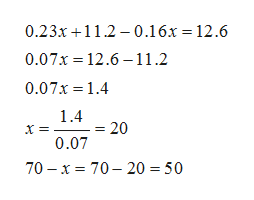A butcher has some hamburger that is 23% fat and some that is 16% fat. How many pounds of each should be mixed to make 70 lb of hamburger that is 18% fat?

Question

A butcher has some hamburger that is 23% fat and some that is 16% fat. How many pounds of each should be mixed to make 70 lb of hamburger that is 18% fat?

Step 1

Let x pounds of hamburger that is 23% fat be used.

Then the number of pounds of hamburger that is 16% fat  used =70-x .

according to given condition , we have

Step 2

On solving the equa...help_outlineImage Transcriptionclose0.23x 11. 0.16x = 12.6 0.07x 12.6 11.2 0.07x 1.4 1.4 20 0.07 70 x 70- 20 = 50 fullscreen

Want to see the full answer?

See Solution

Want to see this answer and more?

Our solutions are written by experts, many with advanced degrees, and available 24/7

See Solution
Tagged in

Equations and In-equations